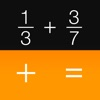# Fraction Calculator + Decimals to Fractions - By Visual Math Interactive Sdn. Bhd. - Lumos Educational App Store4.51
Price -Free
\$0

#### DESCRIPTION:

Introducing the world's first Fraction Calculator packed with additional functions like reducing or simplifying fractions, fractions-to-decimals and a decimals-to-fractions calculator. All these in one nifty app. Discover an easy way to solve everyday fraction problems. Add, subtract, multiply, divide and even convert fractions, quickly and clearly. Visual Math Interactive's Fractions Calculator is an excellent homework help and business reference tool, with nice clean keypads and large display type for your fast and easy calculations. FEATURES: - FREE fraction calculator app and decim

#### OVERVIEW:

Fraction Calculator + Decimals to Fractions - By Visual Math Interactive Sdn. Bhd. is a free educational mobile app By Visual Math Interactive Sdn. Bhd..It helps students in grades 6 practice the following standards 6.NS.1.

This page not only allows students and teachers download Fraction Calculator + Decimals to Fractions - By Visual Math Interactive Sdn. Bhd. but also find engaging Sample Questions, Videos, Pins, Worksheets, Books related to the following topics.

1. 6.NS.1 : Interpret and compute quotients of fractions, and solve word problems involving division of fractions by fractions, e.g., by using visual fraction models and equations to represent the problem. For example, create a story context for (2/3) .

6

#### STANDARDS:

6.NS.1

Developer: Visual Math Interactive Sdn. Bhd.

Software Version: 1.1.8

Category: Productivity

### RELATED APPSEdSearch WebSearch